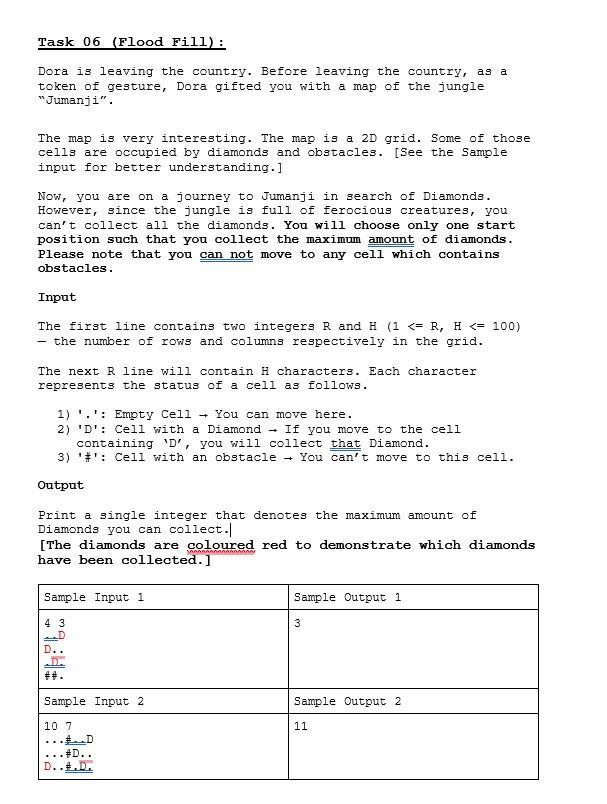# Question must use python   Task 06 (Flood Fill): Dora is leaving the country. Before leaving the country, as a token of gesture, Dora gifted you with a map of the jungle "Jumanji". The map is very interesting. The map is a $$2 \mathrm{D}$$ grid. Some of those cells are occupied by diamonds and obstacles. [See the Sample input for better understanding.] Now, you are on a journey to Jumanji in search of Diamonds. However, since the jungle is full of ferocious creatures, you can't collect all the diamonds. You will choose only one start position such that you collect the maximum amount of diamonds. Please note that you can not move to any celll which contains obstacles. Input The first line contains two integers $$R$$ and $$H(1<=R, H<=100)$$ - the number of rows and columns respectively in the grid. The next $$R$$ line will contain $$H$$ characters. Each character represents the status of a cell as follows. 1) '.': Empty Cell → You can move here. 2) 'D': Cell with a Diamond $$\rightarrow$$ If you move to the cell containing ' $$D^{\prime}$$ ' you will collect that Diamond. 3) '\#': Cell with an obstacle + You can't move to this cell. Output Print a single integer that denotes the maximum amount of Diamonds you can collect.| [The diamonds are coloured red to demonstrate which diamonds have been collected.]GOFC1Y The Asker · Computer Science

must use pythonTranscribed Image Text: Task 06 (Flood Fill): Dora is leaving the country. Before leaving the country, as a token of gesture, Dora gifted you with a map of the jungle "Jumanji". The map is very interesting. The map is a $$2 \mathrm{D}$$ grid. Some of those cells are occupied by diamonds and obstacles. [See the Sample input for better understanding.] Now, you are on a journey to Jumanji in search of Diamonds. However, since the jungle is full of ferocious creatures, you can't collect all the diamonds. You will choose only one start position such that you collect the maximum amount of diamonds. Please note that you can not move to any celll which contains obstacles. Input The first line contains two integers $$R$$ and $$H(1<=R, H<=100)$$ - the number of rows and columns respectively in the grid. The next $$R$$ line will contain $$H$$ characters. Each character represents the status of a cell as follows. 1) '.': Empty Cell → You can move here. 2) 'D': Cell with a Diamond $$\rightarrow$$ If you move to the cell containing ' $$D^{\prime}$$ ' you will collect that Diamond. 3) '\#': Cell with an obstacle + You can't move to this cell. Output Print a single integer that denotes the maximum amount of Diamonds you can collect.| [The diamonds are coloured red to demonstrate which diamonds have been collected.]
More
Transcribed Image Text: Task 06 (Flood Fill): Dora is leaving the country. Before leaving the country, as a token of gesture, Dora gifted you with a map of the jungle "Jumanji". The map is very interesting. The map is a $$2 \mathrm{D}$$ grid. Some of those cells are occupied by diamonds and obstacles. [See the Sample input for better understanding.] Now, you are on a journey to Jumanji in search of Diamonds. However, since the jungle is full of ferocious creatures, you can't collect all the diamonds. You will choose only one start position such that you collect the maximum amount of diamonds. Please note that you can not move to any celll which contains obstacles. Input The first line contains two integers $$R$$ and $$H(1<=R, H<=100)$$ - the number of rows and columns respectively in the grid. The next $$R$$ line will contain $$H$$ characters. Each character represents the status of a cell as follows. 1) '.': Empty Cell → You can move here. 2) 'D': Cell with a Diamond $$\rightarrow$$ If you move to the cell containing ' $$D^{\prime}$$ ' you will collect that Diamond. 3) '\#': Cell with an obstacle + You can't move to this cell. Output Print a single integer that denotes the maximum amount of Diamonds you can collect.| [The diamonds are coloured red to demonstrate which diamonds have been collected.]